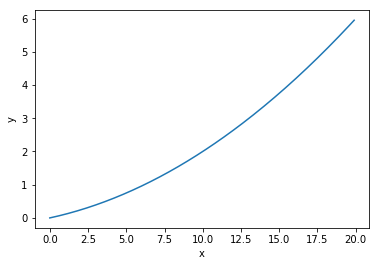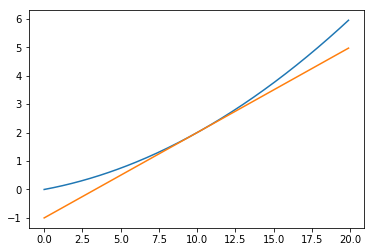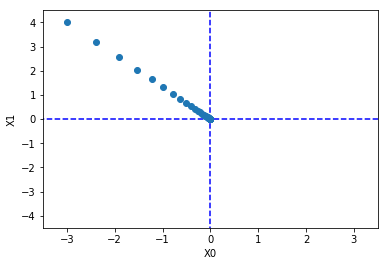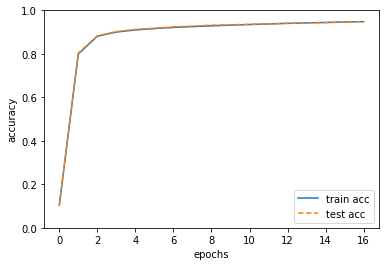# 深度学习之《深度学习入门》学习笔记（四）神经网络的学习

2019/10/4 10:52 上午 posted in  随记

## 损失函数

### 均方误差

$E = \frac{1}{2} \sum_{k}^{}(y_{k}-t_{k})^2$

$$y_{k}$$表示神经网络的输出，$$t_{k}$$表示监督数据，$$k$$表示数据的维度。其中，$$t$$表示监督数据，将正确的解标签设为1，其他均设为0，其他标签表示为0的表示方法称为one-hot表示

# 均方误差定义函数
def mean_squared_error(y, t):
return 0.5 * np.sum((y-t)**2)

# 设“2”为正解
t = [0, 0, 1, 0, 0, 0, 0, 0, 0, 0]

#例1：“2”的概率最高的情况（0.6）
y = [0.1, 0.05, 0.6, 0.0, 0.05, 0.1, 0.0, 0.1, 0.0, 0.0]
mean_squared_error(np.array(y),np.array(t))


0.09750000000000003

# 例2：“7”的概率最高的情况（0.6）
y = [0.1, 0.05, 0.1, 0.0, 0.05, 0.1, 0.0, 0.6, 0.0, 0.0]

mean_squared_error(np.array(y),np.array(t))


0.5975

### 交叉熵误差

$E=-\sum_{k}^{} t_{k} ln y_{k}$

$$y_{k}$$是神经网络的输出，$$t_{k}$$是正确解标签。并且，中只有正确解标签的索引为1，其他均为0（one-hot表示）。交叉熵误差的值是由正确解标签所对应的输出结果决定的。

# 实现交叉熵误差
def cross_entropy_error0(y, t):
delta = 1e-7
return -np.sum(t * np.log(y + delta))


# 进行简单计算
# 设“2”为正解
t = [0, 0, 1, 0, 0, 0, 0, 0, 0, 0]
#例1：“2”的概率最高的情况（0.6）
y = [0.1, 0.05, 0.6, 0.0, 0.05, 0.1, 0.0, 0.1, 0.0, 0.0]
cross_entropy_error0(np.array(y),np.array(t))


0.510825457099338

# 例2：“7”的概率最高的情况（0.6）
y = [0.1, 0.05, 0.1, 0.0, 0.05, 0.1, 0.0, 0.6, 0.0, 0.0]
cross_entropy_error0(np.array(y),np.array(t))


2.302584092994546

### mini-batch学习

$E=-\frac{1}{N} \sum_{n}^{} \sum_{k}^{} t_{nk} ln y_{nk}$

# 读入MNIST 代码暂略
import sys, os
sys.path.append(os.pardir)
import numpy as np

# 使用np.random.choice进行随机选取
np.random.choice(60000, 10)


array([ 5332, 11993, 16553, 47954, 31537, 4750, 52005, 1159, 6775,
46043])

# 可同时处理单个和批量数据
def cross_entropy_error1(y, t):
if y.nidm == 1:
t = t.reshape(1, t.size)
y = y.reshape(1, y.size)

delta = 1e-7
batch_size = y.shape
return -np.sum(t * np.log(y + delta)) / batch_size


# 可同时处理单个和批量数据
def cross_entropy_error2(y, t):
if y.nidm == 1:
t = t.reshape(1, t.size)
y = y.reshape(1, y.size)

delta = 1e-7
batch_size = y.shape
return -np.sum(np.log(y[np.arange(batch_size), t] + delta)) / batch_size


### 为什么要设定损失函数

Q: 为什么要导入损失函数？既然我们的目标是获得识别精度尽可能高的神经网络，那不是应该把识别精度作为指标吗？

A: 在神经网络的学习中，寻找最优参数（权重和偏置）时，要寻找使损失函数的值尽可能小的参数。为了找到使损失函数的值尽可能小的地方，需要计算的参数的导数（确切的讲是梯度），然后以这个导数为指引，逐步更新参数的值。而对权重参数的损失函数求导，表示的是“如果稍微改变这个权重参数的值，损失函数的值会如何变化”。如果导数的值为负如，通过使该权重参数向正方向改变，可以减小损失函数的值；反过来，如果导数的值为正如，则通过使该权重参数向负方向改变，可以减小损失函数的值。当导数的值为0时如，无论权重参数向哪个方向变化，损失函数的值都不会改变，此时该权重参数的更新会停在此处。

## 数值微分

### 导数

Python中的舍入误差：

np.float32(1e-50)


0.0

# 对舍入误差减小与使用中心差分实现函数导数程序
def numerical_diff(f, x):
h = 1e-4 #0.0001
return (f(x+h) - f(x-h)) / (2*h)


### 数值微分的例子

# 实现例子
def function_1(x):
return 0.01*x**2 + 0.1*x

import numpy as np
import matplotlib.pylab as plt

x = np.arange(0.0, 20.0, 0.1) #以0.1为单位，从0到20的数组x
y = function_1(x)
plt.xlabel("x")
plt.ylabel("y")
plt.plot(x, y)
plt.show()#计算上面式子的5,10处导数
numerical_diff(function_1, 5)


0.1999999999990898

numerical_diff(function_1, 10)


0.2999999999986347

def tangent_line(f, x):
d = numerical_diff(f, x)
print(d)
y = f(x) - d*x
return lambda t: d*t + y

x = np.arange(0.0, 20.0, 0.1)
y = function_1(x)
plt.xlabel("x")
plt.ylabel("f(x)")

tf = tangent_line(function_1, 5)
tf2 = tangent_line(function_1, 10)
y2 = tf(x)
y22 = tf2(x)

plt.plot(x, y)
plt.plot(x, y2)
plt.show()
plt.plot(x, y)
plt.plot(x, y22)
plt.show()


0.1999999999990898
0.2999999999986347### 偏导数

# 实现上式的代码
def function_2(x):
#或者return np.sum(x**2)
return x**2 + x**2

# 求偏导1
def function_tmp1(x0):
return x0*x0 + 4.0**2.0

numerical_diff(function_tmp1, 3.0)


6.00000000000378

# 求偏导2
def function_tmp2(x1):
return 3.0**2.0 + x1*x1

numerical_diff(function_tmp1, 4.0)


7.999999999999119

## 梯度

# 梯度的代码实现
h = 1e-4 #0.001

for idx in range(x.size):
tmp_val = x[idx]
# f(x+h)的计算
x[idx] = tmp_val + h
fxh1 = f(x)

# f(x-h)的计算
x[idx] = tmp_val - h
fxh2 = f(x)

grad[idx] = (fxh1 - fxh2) / (2*h)
x[idx] = tmp_val # 还原值



# 求点(3,4) (0,2) (3,0)处的梯度


array([6., 8.])

numerical_gradient(function_2, np.array([0.0, 2.0]))


array([0., 4.])

numerical_gradient(function_2, np.array([3.0, 0.0]))


array([6., 0.])

import numpy as np
import matplotlib.pylab as plt
from mpl_toolkits.mplot3d import Axes3D

h = 1e-4 # 0.0001

for idx in range(x.size):
tmp_val = x[idx]
x[idx] = float(tmp_val) + h
fxh1 = f(x) # f(x+h)

x[idx] = tmp_val - h
fxh2 = f(x) # f(x-h)
grad[idx] = (fxh1 - fxh2) / (2*h)

x[idx] = tmp_val # 还原值

if X.ndim == 1:
else:

for idx, x in enumerate(X):

def function_2(x):
if x.ndim == 1:
return np.sum(x**2)
else:
return np.sum(x**2, axis=1)

def tangent_line(f, x):
print(d)
y = f(x) - d*x
return lambda t: d*t + y

if __name__ == '__main__':
x0 = np.arange(-2, 2.5, 0.25)
x1 = np.arange(-2, 2.5, 0.25)
X, Y = np.meshgrid(x0, x1)

X = X.flatten()
Y = Y.flatten()

plt.figure()
plt.xlim([-2, 2])
plt.ylim([-2, 2])
plt.xlabel('x0')
plt.ylabel('x1')
plt.grid()
plt.legend()
plt.draw()
plt.show()### 梯度法

$x_{0}=x_{0}-\eta \frac{\partial f}{\partial x_{0}}$

$x_{1}=x_{1}-\eta \frac{\partial f}{\partial x_{1}}$

def gradient_descent(f, init_x, lr=0.01, step_num=100):
"""
参数f是要进行最优化的函数，init_x是初始值，lr是学习率learning rate，step_num是梯度法的重复次数。
"""
x = init_x
x_history = []

for i in range(step_num):
x_history.append( x.copy() )

return x, np.array(x_history)

# 用梯度法求f(x0+x1)=x0^2+x1^2的最小值
def function_2(x):
return x**2 + x**2

init_x = np.array([-3.0, 4.0])
x, x_history = gradient_descent(function_2, init_x=init_x, lr=0.1, step_num=100)

# 用图像表示上面的函数梯度下降法的步骤
plt.plot( [-5, 5], [0,0], '--b')
plt.plot( [0,0], [-5, 5], '--b')
plt.plot(x_history[:,0], x_history[:,1], 'o')

plt.xlim(-3.5, 3.5)
plt.ylim(-4.5, 4.5)
plt.xlabel("X0")
plt.ylabel("X1")
plt.show()# 学习率过大的例子：lr=10.0
init_x = np.array([-3.0, 4.0])
x, x_history = gradient_descent(function_2, init_x=init_x, lr=10.0, step_num=100)
x


array([-2.58983747e+13, -1.29524862e+12])

# 学习率过小的例子：lr=1e-10
init_x = np.array([-3.0, 4.0])
x, x_history = gradient_descent(function_2, init_x=init_x, lr=1e-10, step_num=100)
x


array([-2.99999994, 3.99999992])

### 神经网络的梯度

# 一个简单的神经网络
# coding: utf-8
import sys, os
sys.path.append('../input/deeplearningfromscratch/deeplearningfromscratch')  # 为了导入父目录中的文件而进行的设定
import numpy as np
from common.functions import softmax, cross_entropy_error

class simpleNet:
def __init__(self):
self.W = np.random.randn(2,3)

def predict(self, x):
return np.dot(x, self.W)

def loss(self, x, t):
z = self.predict(x)
y = softmax(z)
loss = cross_entropy_error(y, t)

return loss

net = simpleNet()
print(net.W)

x = np.array([0.6, 0.9])
p = net.predict(x)
print(p)

print(np.argmax(p))

t = np.array([0, 0, 1]) # 正确解标签

f = lambda w: net.loss(x, t)

print(dW)


[[ 0.61060921 0.39278321 -0.19230499]
[-0.57160886 0.59945886 -0.26529107]]
[-0.14808245 0.7751829 -0.35414496]
1
[[ 0.13852712 0.34874166 -0.48726878]
[ 0.20779067 0.5231125 -0.73090317]]

## 学习算法的实现

1. mini-batch：从训练数据中随机选出一部分数据，这部分数据称为mini-batch。我们的目标是减小mini-batch的损失函数的值。
2. 计算梯度：为了减小mini-batch的损失函数的值，需要求出各个权重参数的梯度。梯度表示损失函数的值减小最多的方向。
3. 更新参数：将权重参数沿梯度方向进行微小更新。
4. 重复：重复第1、2、3步。

### 2层神经网络的类

from common.functions import *

class TwoLayerNet:

def __init__(self, input_size, hidden_size, output_size, weight_init_std=0.01):
# 初始化权重
self.params = {}
self.params['W1'] = weight_init_std * np.random.randn(input_size, hidden_size)
self.params['b1'] = np.zeros(hidden_size)
self.params['W2'] = weight_init_std * np.random.randn(hidden_size, output_size)
self.params['b2'] = np.zeros(output_size)

def predict(self, x):
W1, W2 = self.params['W1'], self.params['W2']
b1, b2 = self.params['b1'], self.params['b2']

a1 = np.dot(x, W1) + b1
z1 = sigmoid(a1)
a2 = np.dot(z1, W2) + b2
y = softmax(a2)

return y

# x:输入数据, t:监督数据
def loss(self, x, t):
y = self.predict(x)

return cross_entropy_error(y, t)

def accuracy(self, x, t):
y = self.predict(x)
y = np.argmax(y, axis=1)
t = np.argmax(t, axis=1)

accuracy = np.sum(y == t) / float(x.shape)
return accuracy

# x:输入数据, t:监督数据
loss_W = lambda W: self.loss(x, t)

W1, W2 = self.params['W1'], self.params['W2']
b1, b2 = self.params['b1'], self.params['b2']

batch_num = x.shape

# forward
a1 = np.dot(x, W1) + b1
z1 = sigmoid(a1)
a2 = np.dot(z1, W2) + b2
y = softmax(a2)

# backward
dy = (y - t) / batch_num

da1 = np.dot(dy, W2.T)


# 二层神经网络例子
net = TwoLayerNet(input_size = 784, hidden_size = 100, output_size = 10)
print(net.params['W1'].shape)
print(net.params['b1'].shape)
print(net.params['W2'].shape)
print(net.params['b2'].shape)


(784, 100)
(100,)
(100, 10)
(10,)

#推理处理的实现如下
x = np.random.rand(100, 784) # 伪输入数据100笔
y = net.predict(x)
t = np.random.rand(100, 10) # 伪正确解标签10笔



(784, 100)
(100,)
(100, 10)
(10,)

1. __init__(self, input_size, hidden_size, output_size)：类的初始化方法，参数依次表示输入层的神经元数、隐藏层的神经元数、输出层的神经元数。

因为进行手写数字识别时，输入图像的大小是784(28x28)，输出为10个类别，所以指定参数input_size = 784, output_size = 10，将隐藏层的个数, hidden_size设置为一个合适的值即可（例如这里是100）。

2. predict(self, x)、accuracy(self, x, t)同上一章神经网络的推理处理基本一致。

3. loss(self, x, t)是计算损失函数值的方法。这个方法会基于predict()的结果和正确解标签，计算交叉熵误差。

4. numerical_gradient(self, x, t)是计算各个参数的梯度，而gradient(self, x, t)是使用误差反向传播法高效计算梯度的方法。

numerical_gradient(self, x, t)是基于数值微分计算参数的梯度。而gradient(self, x, t)是使用误差反向传播法高速计算梯度，其求到的梯度和数值微分的结果基本一致，且速度比前者快。

### mini-batch的实现

import numpy as np
import matplotlib.pyplot as plt
# from two_layer_net import TwoLayerNet

# 读入数据
(x_train, t_train), (x_test, t_test) = load_mnist(normalize=True, one_hot_label=True)

network = TwoLayerNet(input_size=784, hidden_size=50, output_size=10)

# 超参数
iters_num = 10000  # 适当设定循环的次数
train_size = x_train.shape
batch_size = 100
learning_rate = 0.1

train_loss_list = []
train_acc_list = []
test_acc_list = []

# 平均每个epoch的重复次数
iter_per_epoch = max(train_size / batch_size, 1)

for i in range(iters_num):
# 获取mini-batch

# 计算梯度

# 更新参数
for key in ('W1', 'b1', 'W2', 'b2'):

# 记录学习过程
loss = network.loss(x_batch, t_batch)
train_loss_list.append(loss)

# 计算每个epoch的识别精度
if i % iter_per_epoch == 0:
train_acc = network.accuracy(x_train, t_train)
test_acc = network.accuracy(x_test, t_test)
train_acc_list.append(train_acc)
test_acc_list.append(test_acc)
print("train acc, test acc | " + str(train_acc) + ", " + str(test_acc))

# 绘制图形
markers = {'train': 'o', 'test': 's'}
x = np.arange(len(train_acc_list))
plt.plot(x, train_acc_list, label='train acc')
plt.plot(x, test_acc_list, label='test acc', linestyle='--')
plt.xlabel("epochs")
plt.ylabel("accuracy")
plt.ylim(0, 1.0)
plt.legend(loc='lower right')
plt.show()


train acc, test acc | 0.10441666666666667, 0.1028
train acc, test acc | 0.7994333333333333, 0.8037
train acc, test acc | 0.8809833333333333, 0.8833
train acc, test acc | 0.9003666666666666, 0.9024
train acc, test acc | 0.9098833333333334, 0.9125
train acc, test acc | 0.9164, 0.9178
train acc, test acc | 0.9214, 0.9244
train acc, test acc | 0.92525, 0.9277
train acc, test acc | 0.92875, 0.9321
train acc, test acc | 0.9321166666666667, 0.9337
train acc, test acc | 0.9348333333333333, 0.9358
train acc, test acc | 0.93755, 0.9384
train acc, test acc | 0.9400833333333334, 0.9407
train acc, test acc | 0.9424166666666667, 0.9422
train acc, test acc | 0.9445666666666667, 0.9431
train acc, test acc | 0.9466666666666667, 0.9459
train acc, test acc | 0.9480833333333333, 0.9479epoch是一个单位，一个epoch表示学习中所有训练数据均被使用过一次时的更新次数。